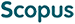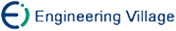陈 军 王国瑾. 利用形状参数构造保凸插值的双曲多项式B样条曲线[J]. 计算机研究与发展, 2006, 43(7): 1216-1224.
 引用本文: 陈 军 王国瑾. 利用形状参数构造保凸插值的双曲多项式B样条曲线[J]. 计算机研究与发展, 2006, 43(7): 1216-1224.Chen Jun and Wang Guojin. Constructing Convexity-Preserving Interpolation Curves of Hyperbolic Polynomial B-Splines Using a Shape Parameter[J]. Journal of Computer Research and Development, 2006, 43(7): 1216-1224.
 Citation: Chen Jun and Wang Guojin. Constructing Convexity-Preserving Interpolation Curves of Hyperbolic Polynomial B-Splines Using a Shape Parameter[J]. Journal of Computer Research and Development, 2006, 43(7): 1216-1224.## Constructing Convexity-Preserving Interpolation Curves of Hyperbolic Polynomial B-Splines Using a Shape Parameter

• 摘要: 把一个参数化的奇异多边形与双曲多项式B样条按某一个因子调配,可自动生成带形状参数且插值给定平面点列的C\+2(或G\+1)连续的双曲多项式B样条曲线.把这一曲线的曲率符号函数写为Bernstein多项式形式,并利用Bernstein多项式的非负性条件,得到形状参数的合适取值来保证样条曲线对插值点列的保凸性.此方法简单、方便,无需解方程组或迭代计算,生成的插值曲线具有较均匀的曲率.大量实例验证了算法的正确与有效.

Abstract: Using a blending factor, a parametrized singular polyline is blended with the hyperbolic polynomial B-spline curve to automatically generate a C\+2 (or G\+1) continuous hyperbolic polynomial B-spline with a shape parameter, which interpolates the given planar data points. By converting the curvature sign function of the interpolating curve into Bernstein polynomial, the nonnegativity conditions of Bernstein polynomial can be used to obtain the appropriate value of the shape parameter satisfying the convexity-preserving property of the constructed curve. The method is simple and convenient and need not to solve a system of equations or recur to a complicated iterative process, and the resulting interpolating curves possess smooth distribution of curvature. Several numerical examples are given to illustrate the correctness and validity of the algorithm./下载:  全尺寸图片 幻灯片
• 分享
• 用微信扫码二维码

分享至好友和朋友圈